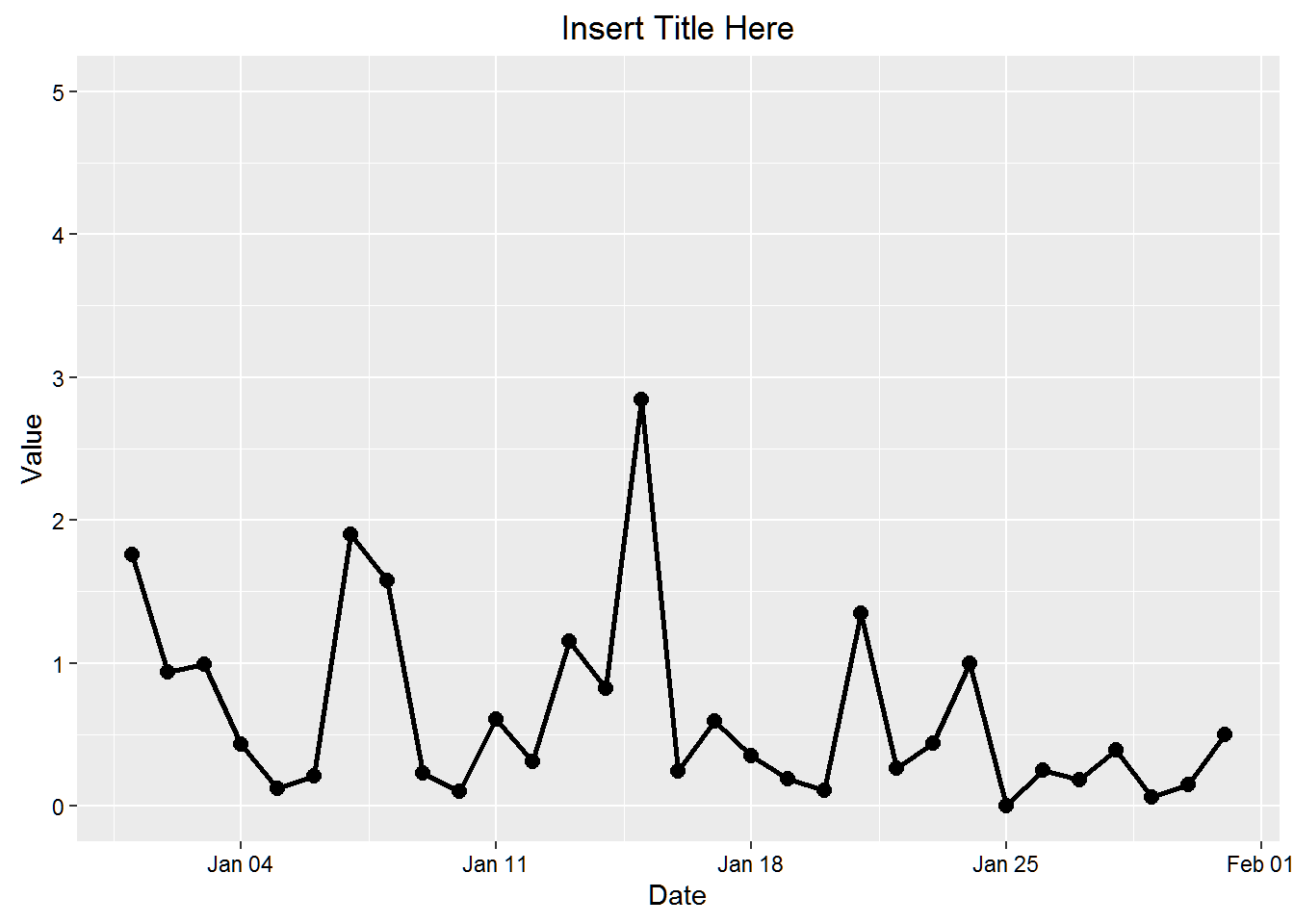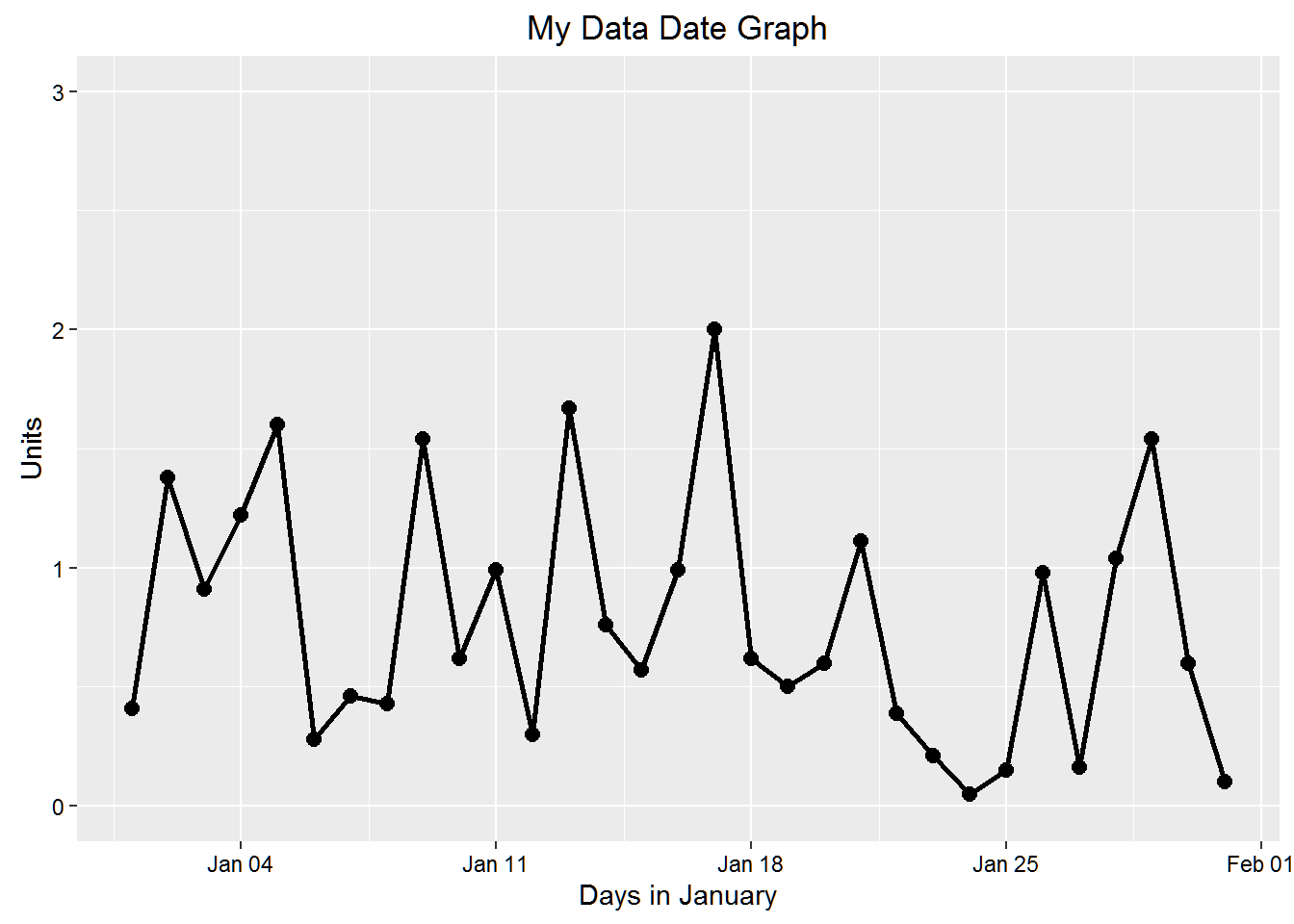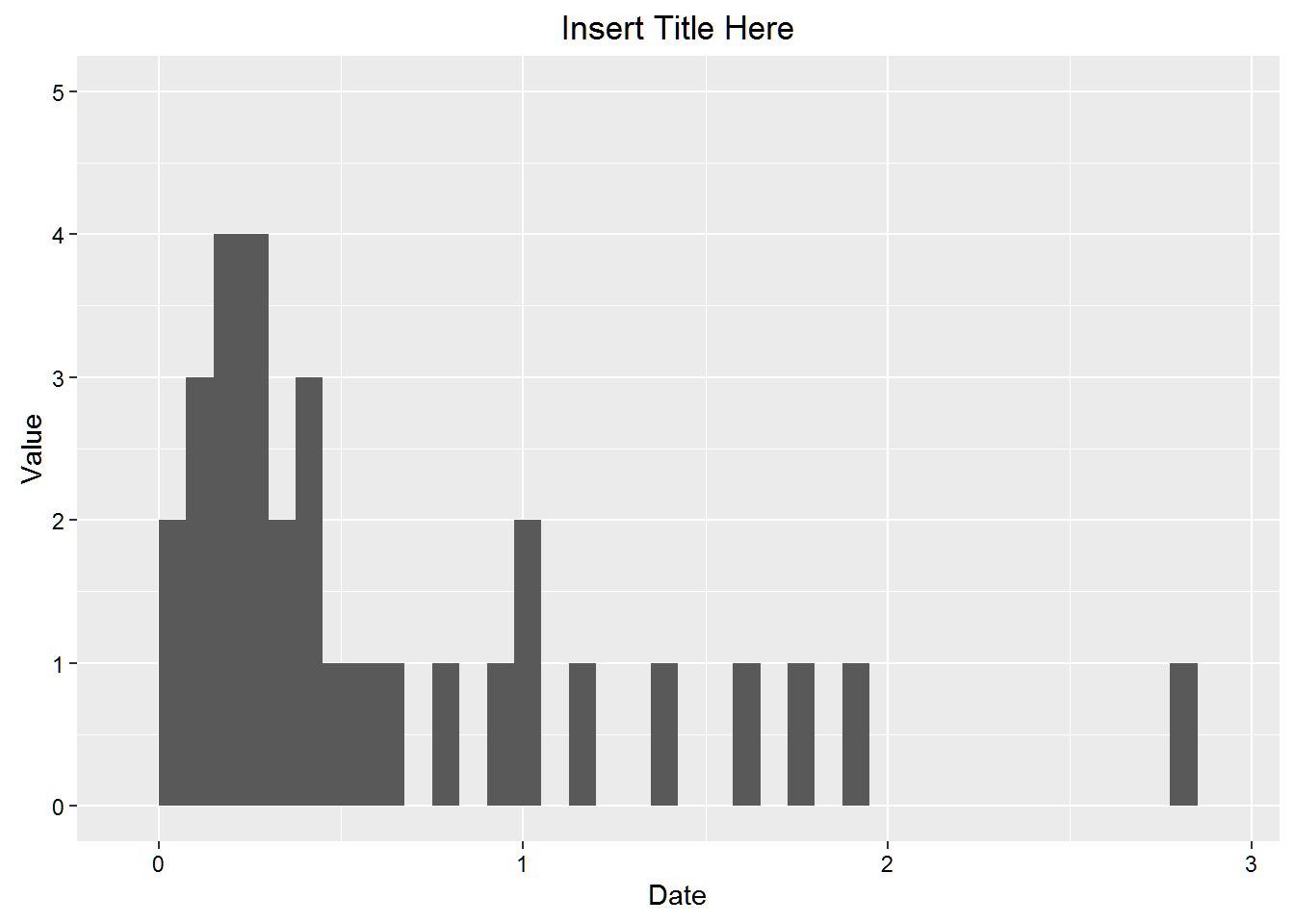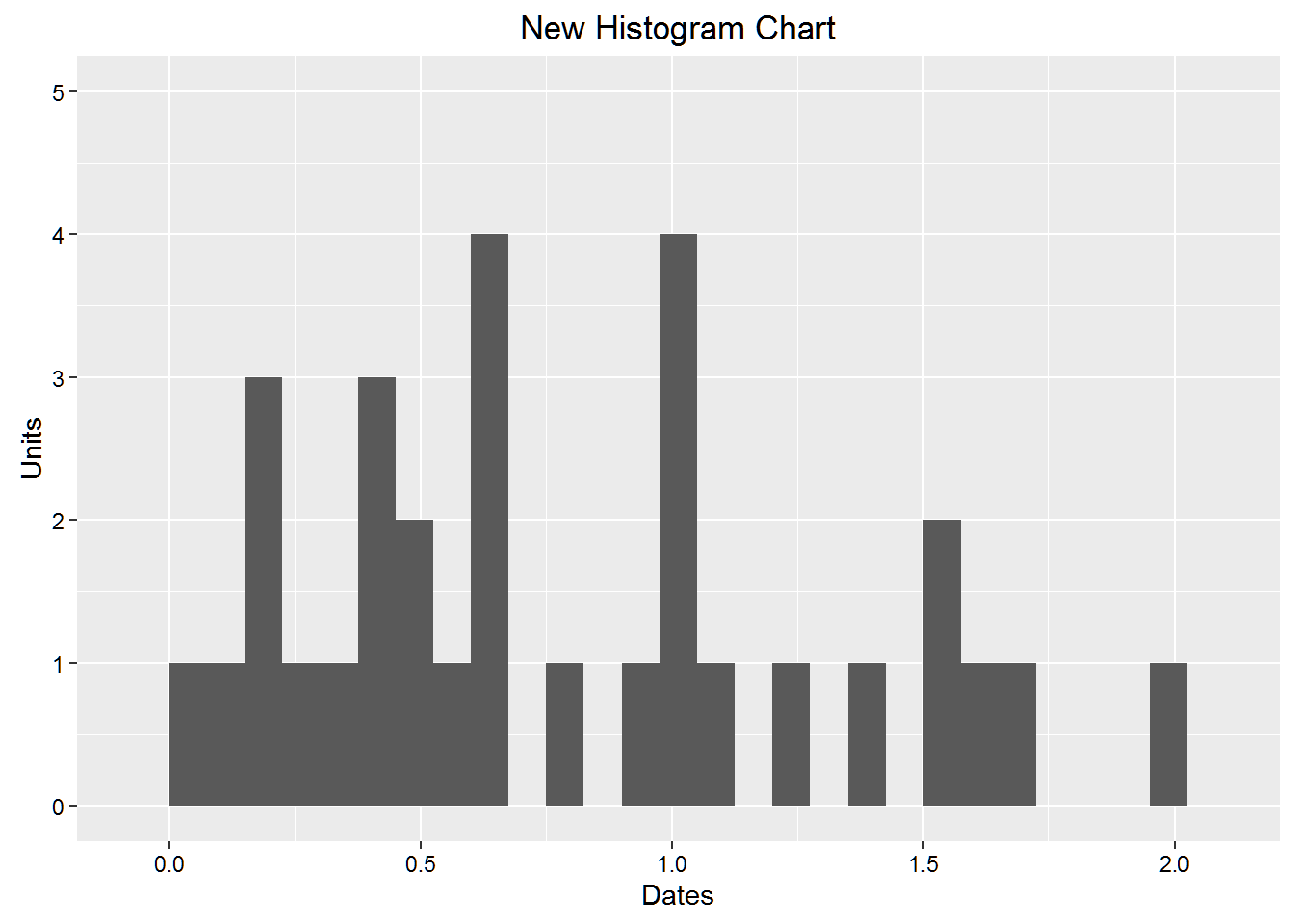## Automating Calls Using ggplot2

One of the duller tasks in R is going through repetitive steps. It is to be expected since R is an interative program. But there out to be a way to make it less dull. That’s what functions are for.

Well, the clever folks at Mathew Analytics show how to automate some of these tasks in the following code.

And here’s the code!

First, create a data frame object.

library(ggplot2)

mydat <- data.frame(date = c(seq(as.Date("2010/01/01"), as.Date("2010/01/31"), by=1)),
value1 = abs(round(rnorm(31), 2)),
value2 = abs(round(rnorm(31), 2)),
value3 = abs(round(rnorm(31), 2)))
head(mydat)
##         date value1 value2 value3
## 1 2010-01-01   1.76   0.88   0.41
## 2 2010-01-02   0.94   0.45   1.38
## 3 2010-01-03   0.99   0.00   0.91
## 4 2010-01-04   0.43   0.17   1.22
## 5 2010-01-05   0.12   0.59   1.60
## 6 2010-01-06   0.21   0.99   0.28

Next, write the function that will end the drudgery.

viz_func <- function(data, x, y){
ggplot(data, aes_string(x=x, y=y)) +
geom_line(lwd=1.05) + geom_point(size=2.5) +
ggtitle("Insert Title Here") +
xlab("Date") + ylab("Value") + ylim(0,5) +
theme(axis.text.x=element_text(colour="black")) +
theme(axis.text.y=element_text(colour="black"))
}

Check our work to see how things are coming along. We should have a nice line chart started.

viz_func(mydat, 'date', 'value1')Let’s add some labels and such.

viz_func(mydat, 'date', 'value3') +
ggtitle("My Data Date Graph") +
xlab("Days in January") + ylab("Units") + ylim(c(0, 3))
## Scale for 'y' is already present. Adding another scale for 'y', which
## will replace the existing scale.You can do something similar with histograms. You need to play around with the binwidth setting because the default is too large for this example. Find a setting that suits your data.

First, the generic version.

viz_func2 <- function(data, x){
ggplot(data, aes_string(x = x)) +
geom_histogram(binwidth = 0.075) +
ggtitle("Insert Title Here") +
xlab("Date") + ylab("Value") + ylim(0,5) +
theme(axis.text.x=element_text(colour="black")) +
theme(axis.text.y=element_text(colour="black"))
}
viz_func2(mydat, 'value1')Now, we snazz it up just a bit. Don’t want to go overboard!

viz_func2(mydat, 'value3') +
ggtitle("New Histogram Chart") +
xlab("Dates") + ylab("Units")